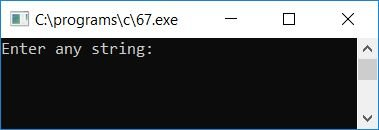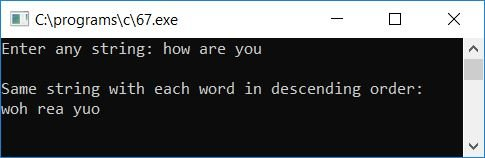# C Program to sort each Word from given String in Descending Order

In this tutorial, we will learn about how to sort or arrange each and every words from any given string (by user at run-time) in descending order. If user supplies any string without spaces, that is only one word as string, then the one word as string will also be arranged in descending order. Here is the program of sorting each word in any given string in descending order.

In this program, Each word is sorted character wise, for example if the given string by user will be this is codescracker. Here we have total of three words present inside the string, therefore we have to sort all the three words inside the string that is this, is and codescracker are the three words to be sorted in descending order. Here after sorting the string, it will become tsih si srrokeedccca. As you can see that, all the three words are sorted or arranged in descending order. Now let's take a look at the program:

```// Write a program in C to sort each word from any
// given string in descending order
// for example, if string is "how are you"
// then after sorting each word in descending order,
// the string has to become "woh rea yuo"
// -----------codescracker.com-----------

#include<stdio.h>
#include<conio.h>
#include<string.h>
int main()
{
char str, chTemp;
int i, j, len;
printf("Enter any string: ");
gets(str);
len = strlen(str);
for(i=0; i<len; i++)
{
for(j=0; j<(len-1); j++)
{
if(str[j]>=65 && str[j]<=90)
{
if(str[j+1]>=65 && str[j+1]<=90)
{
if(str[j]<str[j+1])
{
chTemp = str[j];
str[j] = str[j+1];
str[j+1] = chTemp;
}
}
}
if(str[j]>=97 && str[j]<=122)
{
if(str[j+1]>=97 && str[j+1]<=122)
{
if(str[j]<str[j+1])
{
chTemp = str[j];
str[j] = str[j+1];
str[j+1] = chTemp;
}
}
}
}
}
printf("\nSame string with each word in descending order:\n%s", str);
getch();
return 0;
}```

The program was written under Code::Blocks IDE, therefore after successful build and run, here is the sample run of the above program. This is the first snapshot:Now supply any string say how are you and press ENTER key to see the same string with its each word sorted in descending order as shown here in the snapshot:Here are the list of some main steps used in above program:

• Receive any string using gets() function
• Now find length of the given string using strlen() function of string.h library
• Store the length of given string inside any variable say len
• Create a for loop that starts from 0 to one less than the length of the string to compare its each and every character
• Inside the for loop, create another for loop to run or repeat comparing the string's each and every character
• Inside the second for loop, be confirm that both the character is an alphabet or not, to compare and reverse only when first character is less than the second character or second character is less than the third character and so on.
• Let's suppose if user supplies the string say codescracker, then c is compared with o first, as c is less than p, therefore the reverse operation gets performed, and now the string will become ocdescracker after first check and reverse, now again the second character, that is c is compared with the third character d, here again c is less than d, then again the reverse operation gets performed, and d goes to c's position and c goes to d's position, and then string will become odcescracker and so on
• In this way, all the characters of the string gets sorted in descending order
• Here, we have used two for loop to do comparing and reversing (if previous is less than the next character) two times from start to end
• Finally print the value of string on output screen which will be the same string but arranged in descending order

Tools
Calculator